## Articulo

•Citado por SciELO
•Accesos

•Similares en SciELO

## versión On-line ISSN 1390-6542versión impresa ISSN 1390-9363

### Enfoque UTE vol.9 no.4 Quito oct./dic. 2018

#### http://dx.doi.org/10.29019/enfoqueute.v9n4.393

Automatización y Control,…

Efficient solution of nonlinear model predictive control by a restricted enumeration method

Solución eficiente del control predictivo no lineal basado en modelo mediante un método de enumeración restringida

1 Instituto Tecnológico Metropolitano de Medellín, Facultad de Ingenierías, Medellín - Colombia jhonisaza@itm.edu.co.

2 Optimización Matemática de Procesos ÓPTIMO, Centro de Ciencia Básica, Universidad Pontificia Bolivariana, Medellín - Colombia, diego.munoz@upb.edu.co.

3 KALMAN, Grupo de investigación en Procesos Dinámicos, Universidad Nacional de Colombia, Facultad de Minas, Medellín - Colombia. hdalvare@unal.edu.co.

Abstract:

This work presents an alternative method to solve the nonlinear program (NLP) for nonlinear model predictive control (NMPC) problems. The NLP is the most computational demanding task in NMPC, which limits the industrial implementation of this control strategy. Therefore, it is important to consider algorithms that can solve the nonlinear program, not only in real time but also guaranteeing feasibility. In this work, the restricted enumeration method is proposed as alternative to solve the NLP for NMPC problems, showing successful results for pH control in a sugar cane process plant. This method enumerates in restricted way a set of final control element possible positions around the current one. Next, it tests all positions in that set to find the best one, taken as the optimization solution.

Keywords: restricted enumeration method; nonlinear program (NLP); nonlinear model predictive control (NMPC); pH control.

Resumen:

Este trabajo presenta un método alternativo para resolver los problemas de programación no lineal (NLP) del control predictivo no lineal basado en modelo (NMPC). La NLP es la tarea más exigente en computación en NMPC, lo que limita la implementación industrial de esta estrategia de control. Por lo tanto, es importante tener en cuenta los algoritmos que pueden resolver la programación no lineal, no solo en tiempo real sino también garantizando la viabilidad. En este trabajo, se propone el método de enumeración restringida como alternativa para resolver el problema de la NLP en NMPC, mostrando resultados exitosos para el control del pH en una planta de procesamiento de caña de azúcar. Este método enumera de forma restringida un conjunto de posibles posiciones del elemento final de control alrededor de la posición actual. Luego, prueba todas las posiciones en ese conjunto para encontrar la mejor acción de control, tomada como la solución de optimización.

Palabras clave: método de enumeración restringida; programación no lineal (PNL); control predictivo no lineal basado en modelo (NMPC); control de pH.

1. Introduction

Model predictive control (MPC) is a successful control technique for plants with slow dynamics like chemical and petrochemical processes. Although these kind of plants are nonlinear, in some cases, the performance of linear MPC has been suitable (Darby & Nikolaou, 2012; Mayne, 2000; Rao & Rawlings, 2000; Xi, Li, & Lin, 2013). However, in order to improve the MPC performance a nonlinear model of the process should be used, which leads to nonlinear MPC (NMPC) (Chen & Allgöwer, 1998; De Oliveira, 1996). The main disadvantage of NMPC is the complexity related with the resulting nonlinear optimization problem, which is usually nonconvex (Mishra, 2011) and must be solved in real time (Camacho & Bordons, 2007; Chen & Allgöwer, 1998). A typical solution to solve the resulting nonlinear program (NLP) in real time implies the use of high performance computing, however, in industrial facilities it is not available (Richalet, 1993). Additionally, any required complex computation for control implies an increase in maintenance protocols for which the fail probabilities also increase.

Available numerical methods to solve the non-convex optimization problems for NMPC can be classified in three sub-groups: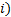Direct enumeration, which is not very efficient at the computation level when the process model is not compact enough, but with models that can be evaluated at a good speed, this technique always produces feasible solutions and quite close to the global optimum;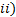dynamic programming, like iterative dynamic programming (Luus, 1996) and blurred dynamic programming (Alkan, Erkmen, & Erkmen, 1994), but the disadvantage of dynamic programming methods is the high computation costs; and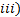heuristic methods such as genetic algorithms, Bacterial Chemotaxis (BCh) and simulated annealing. Although those methods provide feasible solutions very close to the global optimum, they consume a lot of computation time (Holland, 1992). Therefore, to provide simple optimization procedures allows the industrial implementation of NMPC. The aim of this work is to propose the restricted enumeration method to solve the nonlinear-nonconvex optimization problem of NMPC to increase the possibilities of its implementation in industrial processes.

This article is structured as follows. In Section 2, the problem formulation is stated. In Section 3, the optimization by restricted enumeration is proposed. Subsequently, in Section 4 an illustrative case study is presented, showing the suitability of this proposal. Finally, conclusions are given in Section 5.

2. Model predictive control

Model predictive control (MPC) is a control technique applicable to both linear and nonlinear systems, which is based on optimal control theory (Camacho & Bordons, 2007). MPC has its roots in the development of optimal control theory in the fifties of the twentieth century, but achieved its consolidation as an independent technique at the end of the seventies. In this kind of control, a process model predicts the evolution of the variables over a specified finite prediction horizon. Thus, an optimal control strategy is obtained by minimizing an objective function, which usually includes, among others, a term related to the error and another that penalizes the applied control effort, all on a finite prediction horizon. The control action is optimized over a control horizon, which is shorter or at most the same as the prediction horizon. Only the first calculated control action is applied to the process and the optimization is repeated at the next sampling time. Several works have shown that MPC allows the construction of stable control laws (Camacho & Bordons, 2007; Chen & Allgöwer, 1998; De Oliveira, 1996; Xi et al., 2013).

For linear system and quadratic objective function, MPC strategies are considered as well-founded subject in which stability, optimality and feasibility of control actions are guaranteed (Camacho & Bordons, 2007). In contrast, non-linear MPC arises when the prediction model is nonlinear and/or restrictions on the states of the process are included into the optimization problem. In this case, a nonlinear optimization problem must be efficiently solved to obtain the control actions. Those control actions should fulfill certain characteristics, including their feasibility. For nonlinear systems, the nonlinear MPC can be formulated as shown in Equations 1a - 1e.

Where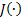, Equation (1a), is the control performance objective function,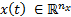denotes the vector of state variables andis the vector of manipulated inputs or calculated control actions. Process model is represented by Equation (1b) with corresponding initial conditions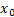, Equation (1c), while Equation (1d)-(1e) are equality and inequality constraints for the process, respectively. The vector fields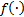,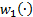, and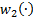map from some open subsets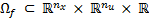and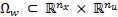into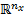,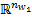, and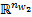, respectively, and are assumed to be continuous in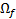and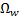, respectively.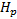is the prediction horizon and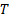the sampling period.

In general,is expressed as shown in Equation 2.

Where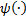is a penalty function based on initial and terminal conditions of the system and integral terms evaluate the state transient behavior of the system for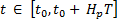and the control action behavior for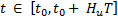with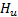the control horizon.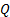and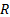are positive definite matrices acting as weights for representing the biased solution between minimum error in process output but reduced control effort.

The basic MPC structure used in this paper is shown in Figure 1, where it is assumed that the complete state vector is available from direct measurements at the plant. This assumption was considered to avoid the implementation of a state estimator and to make easier the validation of the proposed optimization methodology. However, the use of this proposal with one or more estimators for those non-measured states is direct.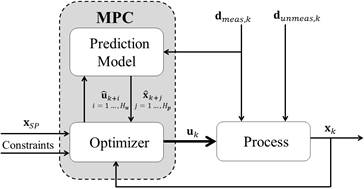Fig. 1: Model predictive control structure. The state vector of the plant is assumed completely available.

As shown in Figure 1, the prediction model uses both input (manipulated and disturbances) and output information (states as measured variables) to compute the future estimated states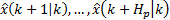for a future horizon. At sampling time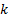, the MPC given by Equation (1), predicts the set of control actions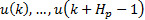, which allow that the states track a predefined trajectory over the prediction horizon. This is possible with the manipulated variable executing the computed movements in the control horizon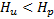and guaranteeing feasibility respect to equality, Equation (1d), and inequality, Equation (1e), constraints. From time instantto time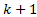, only the first element of the computed sequence of manipulated variables is applied to the process. At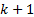all the measurements are actualized allowing the prediction model to use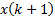and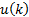to compute the future state vector. The prediction horizonand control horizonmove a step forward, which is called moving horizon control.

The solution of the optimization problem is the part of the NMPC algorithm with the highest computational cost, in particular when the considered model is non-linear and constraints are included. To this aim, in a general approach three kind of numerical methods can be used:Direct enumeration,Dynamic programming methods (Alkan et al., 1994; Aydin, Bonvin, & Sundmacher, 2017; Binder, Cruse, Villar, & Marquardt, 2000; Holland, 1992), andHeuristics methods. In this work, a direct enumeration method is proposed, which consists in the generation of possible control vectors to find a minimum value for the NMPC objective function. This control vectors are the position of the FCE during the prediction horizon Hp. It should be notice that the attained “degree of optimality” of the problem depends on the amount of control vectors that are generated. Under these circumstances, there are three different techniques to generate possible control vectors: Random, staggered and restricted enumeration. Random and staggered techniques chose the control vectors without knowledge of the process, while the restricted enumeration not only includes possible positions of actuator but also takes into account the dynamic response of the used final control element (FCE). The construction of this set of FCE movements imitates the branch and bound technique to solve decision trees used in Artificial Intelligence (AI). In this way, a drastic and organized reduction of the search space is obtained, and the time for solving the NMPC optimization problem is reduced compared to direct enumeration methods. Thus, the method of generating control vectors by restricted enumeration allows an organized and successful choice of the “near to best” control action at each sampling time unlike the other two previous methods (random and staggered). This technique is considered in this work and described in the following section.

3. Optimization by restricted enumeration

Optimization by restricted enumeration (ORE) is a numerical method to solve nonlinear optimization problems. Some previous works report similar approaches to other tasks not related to MPC (Vatter, 2008) and (Zenem, Chehade, & Yalaoui, 2012). As it is known, the major obstacles to implement model predictive control (MPC) at industrial environments are two: the availability of a precise enough process model and the computational burden of optimization into MPC algorithm. The model availability is being solved with increasingly powerful modeling techniques. However, for reducing the optimization computational burden, it is necessary to try new approaches. In this sense, the ORE approach proposed here offers a set of possible solutions to the optimization problem. It should be highlighted that mentioned enumeration is non-exhaustive, i.e., only a part of all possible solutions are tested. The real movement capability of the final control element (FCE) is used as the criterion to perform the enumeration among all possible and feasible solutions. Compared to heuristic and gradient-based optimization methods, ORE is a technique with less computation effort and inherent convergence. Its application to MPC optimization solving was not found in the literature as a single topic. Additionally, ORE takes into account the characteristics of the FCE with a direct and simple industrial implementation. According to (Alvarez, 2000), ORE requires the following steps, which are modified in this paper for including the option of control horizongreater than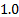:

Create a grid step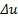from the current position of the FCE acting over the manipulated variable, such that (Equations 3a - 3c):

whereis selected as an enough fine and feasible FCE movement with detectable effect over process state. Note that coarsevalues will produce non-smooth state changes. Contrary, very finevalues will produce excessive computational burden and possibly the FCE will be unable to execute those fine movements. The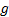subindex indicates the grid current range of FCE incharged of execute the control action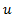. This generates the new dynamic restriction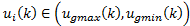, with the limits calculated as:

with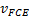the operative speed of FCE in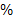of movement per second,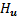the number of movements considered for the FCE during optimization (called control horizon) andthe time step of NMPC in seconds. The difference between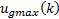and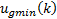is called the restricted range of enumeration. This is the reason to call the current proposal as restricted enumeration, contrary to the known total enumeration.

Eliminate from previous found grid all control actions out of feasible FCE range. In this way it is guaranteed that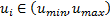, with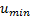and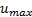the extremes of real FCE, i.e., negative positions or FCE positions over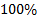are not considered.

Test one to one each position of the final feasible stated grid around the current FCE position. Select as the best feasible control actions sequence (CAS)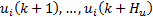, which maxims the improvement of objective function for the MPC, Equation (1). The selected CAS is the solution to the optimization problem, which is feasible but not necessarily a local or global minimum.

Note that the ORE method requires to tune only one parameter, namely, because the other parameters:,, and, are known from FCE coupling with the process and from FCE manufacturer manual.andare time-dependent and must be calculated at eachtime, as it was shown. The option of one-step ORE and multi-step ORE is possible after modification proposed above regarding the original proposal. Next, these two options are exposed.

3.2 Multi-step ORE (H U >1)

Contrary to previous option, multi-step ORE changes the control action during two or more steps into the prediction horizon, in accordance with the control horizon value. Each FCE movement is calculated following the three-step procedure given above. In Figure 3 this option is illustrated for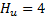. In the same way that applied for one-step ORE, here after the fourth FCE movement the rest of FCE positions are keeped constant and equal to the last one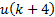, until reach the end of the.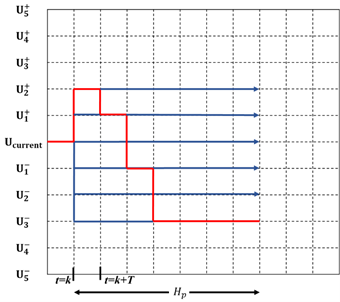Fig. 3: MPC operation using multi-step ORE with four steps forward (). In red the optimal control action sequence found after optimization executed.

Multi-step ahead approach to implement ORE suffers of the curse of dimensionality. For example, trying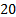possible control sequences with three-step ahead control horizon, the number of option to be tested is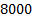control actions combinations. Obviously, this is very time consuming requiring strategies to reduce that explosion of options to be tested. Among that strategies, the most easy to use and enough effective to reduce the number of combinations is to propose valve movements favoring the process error reduction. In that way a half of options is immediately reduced. If the same criterion is applied over the future errors detected by prediction with the model into the prediction horizon, a bigger reduction in the options to be tested is reached. That strategy is the used here only to determine the time reduction in the optimization time during NMPC execution.

4. Illustrative case study

Currently, most of the industries deal with a changing market which is difficult to predict. The consumers demand better characteristics and specifications of products, while the companies try to improve performance and profitability indexes. In addition, the processing of agricultural commodities implies the variability of raw materials regarding the interesting compound. In spite of these situations, the final product must have the same quality. For this purpose, it is necessary to implement advanced control strategies for industrial processes that operate at multiple operating points and achieve optimal conditions.

The case study considered in this work corresponds to the pH control of sugar cane juice in the sugar industry. pH is an important variable to be tracked in order to keep the standard of final sugar. The aim is to control the pH in the alkalinization section of a sugar cane plant. Alkalinization is a part of juice clarification sub-process, but affecting subsequently processes as evaporation and crystallization. During alkalinization, the juice is added with a lime slurry to compensate the pH after sulphitation process, as it is illustrated in Figure 4. The purpose of this process is to neutralize the juice todealing with the highly nonlinear and complex dynamics inherent to pH variable. Note that these facts difficult the use of conventional control systems as PID.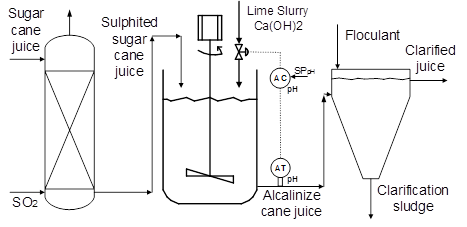Fig. 4: Alkalinization section of a sugar cane plant for pH control.

For controlling this process, it is used the phenomenological based semi-physical model (PBSM) developed in (Isaza & Alvarez, 2011) for the alkalinization stage of the sugar cane plant La Unión S.A, located in Guatemala. In this model, the variables are classified as follows: the controlled variable is the pH of the alkalized juice, the manipulated variable is the flow of lime slurry, also called whitewash, and the most common disturbances in the process are the flow of sulphited juice, the pH of sulphited juice and the pH of lime slurry, which changes with the aging of the slurry. Table 1 shows the operating ranges of the variables involved in the process.

For the simulation study, three disturbances are considered: the reduction in the flow of sulphited juice at 1 minute after beginning at stationary state, the reduction in the pH of sulphited juice at 6 minutes, and the increase of pH of lime slurry at 12 minutes, as shown in Figure 5. These disturbances are commonly found at the sugar cane clarification process in the real plant. This fact justify their use here.

Table 1 Variables classification and operation conditions of alkalinization section of a sugar cane plant.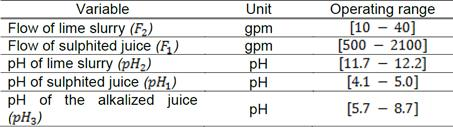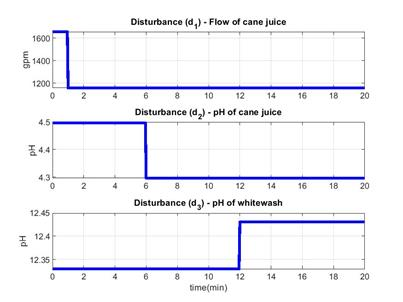Fig. 5: Disturbances applied over the process: flow of sulphited juice, the pH of sulphited juice and the pH of lime slurry.

In order to reject these disturbances, a PID controller tuned using the technique presented in (Isaza & Alvarez, 2011) is used as the first option (the initially installed in that process). The performance of this controller faced with mentioned disturbances is shown in Figure 6. The pH behavior is oscillatory without stay over the set point during all the time. The disturbances effect is immediately reflected on pH as changes on oscillation amplitude. In the same way, the final control element output is oscillatory too, causing high mechanical stress to the valve dosing the lime slurry. It should be highlighted that negative flows of lime slurry were accepted in simulation to maintain unchanged the simulation program. Obviously, an IF structure put in the code conduce to the saturation of controller output at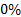with the real consideration of those flow conditions are unfeasible in a real process. Several tuning alternatives where tested to improve the PID response, being the presented in this figures the best one obtained, indicating that Derivative effect is put in the control at its lower value (0.016), only to maintain the full PID structure. In addition a filtering of the pH signal is applied to reproduce the built-in electronic filter of the used pH transmitter (Hanna Instruments).

As it can be seen, the PID response is poor due to its inability to predict the typical abrupt changes on pH response, totally caused by the strong nonlinear behavior of this variable when small disturbances appear. That poor response of PID loop leads to think in using a NMPC as a feasible strategy to improve the pH control performance. Here, a NMPC strategy using the optimization algorithm proposed in this work, namely ORE, is illustrated. In spite of proofs with ORE using control horizon greater than, the simulation results presented here are for control horizon of. The nonlinear PBSM is used not only as the predictor for the NMPC but also to simulate the process response. This is not a limitation because the model is used in its complete version or with all its nonlinear characteristics in both tasks. Figures 7 and 8 correspond to the pH variable and the movements of the valve dosing the lime slurry or whitewash flow, respectively, both using the NMPC using ORE with control horizon equal to.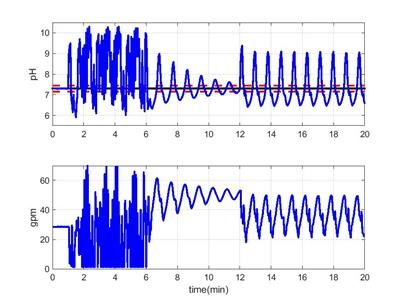Fig. 6: Time evolution of pH and control variable for the alkalinization section of a sugar cane plant.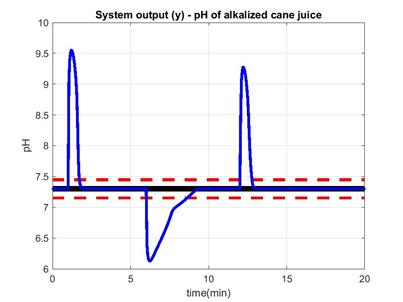Fig. 7: Time evolution of controlled variable: pH of alkalized juice.

It is evident the better response of the process when the NMPC is used compare to PID response. The pH behavior is less oscillatory with only the typical overshoot produced by the first effect of disturbance. After that, the prediction ability of the NMPC compensates the disturbance long term effect maintaining the pH at the stated set point (SP). The final control element (FCE) movements are smooth and sequentially reached, due to the optimization by restricted enumeration used to solve the optimization problem of NMPC. The evident conclusion is the small mechanical stress to which the control valve is subjected. This fact, in addition to the better controlled response indicates the superiority of the NMPC over conventional PID control strategy, as it is expected. The ideal situation is to compare the current proposal with an industrial MPC structure. However, here the comparison was done regarding a PID controller because this kind of controller is the current one installed in the real process.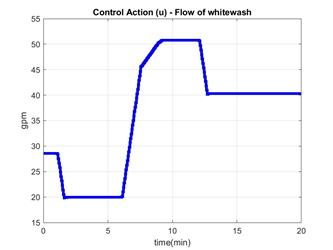Fig. 8: Time evolution of manipulated variable: flow of lime slurry.

Regarding computational cost of NMPC solved with ORE vs. NMPC solved with known optimization algorithms, a test was done indicating a reduction of near to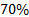of computational cost for optimization executed using Matlab code. This results is for ORE with only one-step ahead or control horizon equal to. For control horizon of three, the reduction in computation time is less significant, being of only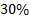. However, using a control horizon ofthe performance of NMPC is the presented in previous results, which is enough to prefer ORE regarding other optimization approaches. In addition, the program to execute ORE with one-step ahead is as simple as it could be written with little lines of code in typical PLC platform. Its main characteristic is the absence of elaborated calculations to execute the optimization with the only requirement of solving the nonlinear PBSM of the process for different input or control action values. In that sense, other option is to convert the PBSM in format of differential and algebraic equations to a fuzzy inference systems, particularly Takagi-Sugeno as it is illustrated in (Isaza & Alvarez, 2011). Using that approach, the computation time is drastically reduced because to solve a Takagi-Sugeno FIS only require the basic mathematical operations. All these facts indicate the high applicability of ORE at industrial environments when the computational hardware is limited.

5. Conclusions

The main restriction of the industrial use of advanced control techniques is the complexity and robustness of their algorithms. From the practical point of view, the optimization by restricted enumeration (ORE) method was implemented showing feasible results. However, the most important contribution of the ORE method is its algorithmic simplicity, which is easily programmable in industrial control equipment such as a PLC. Thus, industrial implementation of advanced control techniques based on optimization is enhanced.

As a future work to extend the control horizon for multi-steps ORE, the number of possible combinations that this method requires to solve the optimization problem must be reduced. A predictive controller with enough dynamic information of the near future of the plant will have more possibilities to correct errors or future disturbances. In other words, it is not the same to excite the prediction model once and to keep the control action value during the prediction horizon, than to excite the model with a variable control action, which allows the model to exhibit dynamic characteristics of interest such that there is greater predictability on future plant behavior.

References

Alkan, M., Erkmen, A. M., & Erkmen, I. (1994). Fuzzy dynamic programming. In Proceedings of MELECON ’94. Mediterranean Electrotechnical Conference (pp. 723-726). IEEE. https://doi.org/10.1109/MELCON.1994.380904Links ]

Alvarez, H. (2000). Control predictivo basado en modelo borroso para el control de pH (Temas de Automática, 10). Editorial Fundación UNSJ, San Juan, Argentina. [ Links ]

Aydin, E., Bonvin, D., & Sundmacher, K. (2017). Dynamic optimization of constrained semi-batch processes using Pontryagin’s minimum principle - An effective quasi-Newton approach. Computers & Chemical Engineering, 99, 135-144. [ Links ]

Binder, T., Cruse, A., Villar, C. A. C., & Marquardt, W. (2000). Dynamic optimization using a wavelet based adaptive control vector parameterization strategy. Computers & Chemical Engineering , 24(2), 1201-1207. [ Links ]

Camacho, E. F., & Bordons, C. (Carlos). (2007). Model predictive control. Springer. [ Links ]

Chen, H., & Allgöwer, F. (1998). Nonlinear Model Predictive Control Schemes with Guaranteed Stability. In Nonlinear Model Based Process Control (pp. 465-494). Dordrecht: Springer Netherlands. https://doi.org/10.1007/978-94-011-5094-1_16Links ]

Darby, M. L., & Nikolaou, M. (2012). MPC: Current practice and challenges. Control Engineering Practice, 20(4), 328-342. [ Links ]

De Oliveira, S. L. (1996). Model predictive control (MPC) for constrained nonlinear systems . Retrieved from https://thesis.library.caltech.edu/5069/Links ]

Holland, J. H. (1992). Adaptation in natural and artificial systems : an introductory analysis with applications to biology, control, and artificial intelligence. MIT Press. [ Links ]

Isaza, J. A., & Alvarez, H. (2011). Selección de parámetros para un sistema de inferencia borrosa Takagi-Sugeno con conjuntos borroso multidimensionales. In Proceedings of XIV Reunión de Trabajo en Procesamiento de la Información y Control RPIC. [ Links ]

Luus, R. (1996). Numerical Convergence Properties of Iterative Dynamic-Programming When Applied to High-Dimensional Systems. Chemical Engineering Research & Design, 74(1), 55-62. [ Links ]

Mayne, D. Q. (2000). Nonlinear Model Predictive Control. In F. Allgöwer & A. Zheng (Eds.) (pp. 23-44). Birkhäuser. [ Links ]

Mishra, S. K. (Ed.). (2011). Topics in Nonconvex Optimization (Vol. 50). New York, NY: Springer New York. https://doi.org/10.1007/978-1-4419-9640-4Links ]

Rao, C. V., & Rawlings, J. B. (2000). Linear programming and model predictive control. Journal of Process Control, 10(2-3), 283-289. https://doi.org/10.1016/S0959-1524(99)00034-7Links ]

Richalet, J. (1993). Industrial applications of model based predictive control. Automatica, 29(5), 1251-1274. https://doi.org/10.1016/0005-1098(93)90049-YLinks ]

Vatter, V. (2008). Enumeration Schemes for Restricted Permutations. Combinatorics, Probability and Computing, 17(1), 137-159. https://doi.org/10.1017/S0963548307008516Links ]

Xi, Y. G., Li, D. W., & Lin, S. (2013). Model Predictive Control - Status and Challenges. Acta Automatica Sinica, 39(3), 222-236. [ Links ]

Zenem, Y. O., Chehade, H., & Yalaoui, A. (2012). New restricted enumeration method for production line design optimization. In IFAC Proceedings Volumes (Vol. 45, pp. 1347-1352). [ Links ]

Received: May 15, 2018; Accepted: October 30, 2018This is an open-access article distributed under the terms of the Creative Commons Attribution License